# Parameters: Transient Solver

Model ElementParam_Transient defines the simulation control parameters for a time-domain-based nonlinear dynamic analysis.

## Description

These parameters control:
• The choice of the integrator.
• The integration error tolerance (or the accuracy of the solution).
• Integrator performance during the simulation.

Dynamic simulations are performed on systems with one or more degrees of freedom. The dynamic simulation accounts for all inertia effects, all applied forces, and internal constraints. This enables you to run accurate system level simulations of complex mechanical systems.

Param_Transient supports two different categories of equation formulations:
• The Ordinary Differential Equations (ODE) form of the equations.
• The Differential Algebraic Equations (DAE) form of the equations.

The settings for each formulation are documented separately.

## Format

ODE Form DAE Form
<Param_Transient

[ integrator_type = "string" ]

[ integr_tol = "real" ]

[ h_max = "real" ]

[ h0_max = "real" ]

[ vel_tol_factor = "real" ]

[ max_order = "integer"]

[ rel_abs_tol_ratio = "real" ]

/>
<Param_Transient

[ integrator_type = "string" ]

[ integr_tol = "real" ]

[ h_max = "real" ]

[ h_min = "real" ]

[ h0_max = "real" ]

[ vel_tol_factor = "real" ]

[ max_order = "integer"]

[ dae_alg_tol_factor = "real" ]

[ dae_index = "integer"]

[ dae_constr_tol = "real" ]

[ dae_corrector_maxit = "real" ]

[ dae_corrector_minit = "real" ]

[ dae_vel_ctrl = "TRUE|FALSE"]

[ dae_jacob_eval = "integer"]

[ dae_eval_expiry = "integer"]

[ dae_jacob_init = "integer"]

[ dae_interpolation = "TRUE|FALSE"]

/>

## Attributes (ODE)

integrator_type
Defines the integrator to be used with the ODE form of the equations of motion. Select one of the following:
• VSTIFF
• MSTIFF
• ABAM
The default value for integrator_type is VSTIFF. See the Comments below for a more detailed explanation of these integrators.
integr_tol
Represents the maximum absolute error per step that the integrator is allowed in computing the displacement, velocity, and differential equations states. Differential equation states include those found in <Control_Diff>, <Control_SISO> and the <Control_StateEqn> modeling entities.
Since displacement and velocity have different units, they are subject to different error tolerances.
• Displacement tolerance = integr_tol
• Velocity tolerance = vel_tol_factor*integr_tol
• Differential equation state tolerance = integr_tol
The default value for integr_tol is 1.0E-3.
vel_tol_factor is a scale factor that you specify separately.
h_max
Defines the maximum step size the integrator is allowed to take. You can also use this parameter to control the accuracy of the results. Generally, a smaller time step leads to more accurate results. For models with discontinuities, such as contact, a smaller value of h_max should be specified. Note that the maximum integrator step size is set to the print_interval in the Simulate command if the print_interval is smaller than h_max.
The default value for h_max is 1.0E-3.
h_min
Defines the minimum step size the integrator is allowed to take.
The default value for h_min is 1.0E-6.
h0_max
The maximum initial step size. The default value for h0_max is 1.0E-8.
vel_tol_factor
A factor that multiplies integr_tol to yield the error tolerance for velocity states.
The default value for vel_tol_factor is 1000. A good value for vel_tol_factor can generally be calculated as 1/h, where h is the dominant step size for your simulation. To try and reduce the analysis time, a value larger than the default 1000 may be used. However, this will reduce accuracy in the velocity states of your model.
rel_abs_tol_ratio
The factor that multiplies integr_tol to yield the relative error tolerance for the integrator.
The default value is 0.01.
max_order
Specifies the maximum order that the integrator is to take. Each integrator has its own built-in maximum order. Higher orders lead to higher accuracy, but lower stability in the numerical method. The table below shows the maximum order for the various integrators in MotionSolve that work with the ODE form of the equations.
Integrator
Maximum Order
ABAM
12
VSTIFF
05
MSTIFF
09
For models that contain contact or other discontinuous events, it may beneficial to set max_order = 2.

## Attributes (DAE)

integrator_type
Defines the integrator to be used with the DAE form of the equations of motion. Currently, only one DAE integrator is supported - DSTIFF.
See the Comments section below for a more detailed explanation of this integrator.
integr_tol
Represents the maximum absolute error per step the integrator is allowed in computing the displacement, velocity, and differential equations states. Differential equation states include those found in <Control_Diff>, <Control_SISO>, and the <Control_StateEqn> modeling entities.
Since displacement and velocity have different units, they are subject to different error tolerances.
• Displacement tolerance = integr_tol
• Velocity tolerance = vel_tol_factor*integr_tol
• Differential equation state tolerance = integr_tol
The default value for integr_tol is 1.0E-3.
vel_tol_factor is a scale factor that you specify separately.
h_max
Defines the maximum step size the integrator is allowed to take. You can also use this parameter to control the accuracy of the results. Generally, a smaller time step leads to more accurate results. For models with discontinuities, such as contact, a smaller value of h_max should be specified.
The default value for h_max is 1.0.
h_min
Defines the minimum step size the integrator is allowed to take.
The default value for h_min is 1.0E-6.
h0_max
The maximum initial step size.
The default value for dae_h0_max is 1.0E-3.
vel_tol_factor
The factor that multiplies integr_tol to yield the error tolerance for velocity states.
The default value for vel_tol_factor is 1000. A good value for vel_tol_factor is the highest frequency in the system that you want to track.
max_order
Specifies the maximum order that the integrator is to take. Higher orders lead to higher accuracy, but lower stability in the numerical method. The default maximum order for DSTIFF is 5.
For models that contain contact or other discontinuous events, it may be beneficial to set max_order = 2, which prevents the integrator from trying to use a higher order that will normally not succeed.
dae_alg_tol_factor
The factor that multiplies integr_tol to yield the error tolerance for algebraic states like Lagrange Multipliers.
The default value for dae_alg_tol_factor is 1000.
dae_index
The index of the DAE formulation.
Index 3 indicates that position constraints are added to the equations of motion.
Index 1 indicates that position, velocity, and acceleration constraints are added (Stabilized Index 1).
See Comments 2-3 for more detailed information.
The default value for dae_index is 3.
dae_constr_tol
The tolerance on all algebraic constraint equations that the corrector must satisfy at convergence.
The default value for dae_constr_tol is 1.0E-5. Decrease this value only if you see a lot of "noise" in the joint or motion reaction forces. Smaller values for this attribute will decrease the speed of the solution. 12
dae_corrector_maxit
The maximum number of iterations that the corrector is allowed to take to achieve convergence.
The default value for dae_corrector_maxit is 4. Under no circumstances should this be increased beyond 8. If the results don't converge for a particular time step, it may be better to let the integrator fail and try to solve with a smaller time step.
dae_corrector_minit
The minimum number of iterations that the corrector is allowed to take before it checks for corrector divergence.
The default value for dae_corrector_minit is 0. A value of 0 lets the integrator choose the minimum number of iterations. The integrator may choose to skip corrector iterations if the norm is less than the tolerance for a given step.
Under no circumstances should this be increased beyond 3.
dae_vel_ctrl
The logical flag that controls whether the velocity states are checked for local integration error at each step. This is only valid for the SI1 integrator (see dae_index).
• "TRUE" indicates that the integrator is to include velocity states in its check for local integration at the end of each step.
• "FALSE" indicates that the integrator is to exclude velocity states in its check for local integration at the end of each step.
When not specified, this defaults to "TRUE" for the SI1 formulation and "FALSE" for the I3 formulation. For more information on formulations, refer to comments 4-6.
Integrator
DAE_VEL_CTRL
I3 Formulation
"FALSE"
SI1 Formulation
"TRUE"
The I3 formulation is not designed to track velocity errors. Hence, even when DAE_VEL_CTRL is "TRUE" for I3, velocity errors will not be tracked.
dae_jacob_eval
This attribute controls the frequency of evaluation of the Jacobian matrix during corrector iterations.
0
Implies that the integrator automatically determines when a new Jacobian is needed by examining the rate of convergence.
1
Implies that a new Jacobian is to be calculated at every iteration.
2
Implies that a new Jacobian is to be calculated at every other iteration, for example at iteration 1-3-5, and so on.
3
Implies that a new Jacobian is to be calculated at every third iteration, for example at iterations 1-4-7, and so on.
Specify dae_jacob_eval only when the default setting does not work well. Frequent Jacobian iterations can slow down the simulations dramatically.
When not specified, the integrator automatically determines when a new Jacobian is needed by examining the rate of convergence.
The Jacobian evaluation is also affected by the attribute dae_jacob_init. 13
dae_eval_expiry
This is an optional attribute. It defines the number of integration steps after which the evaluation pattern defined by dae_jacob_eval is ignored, and the default evaluation pattern is to be used. The default evaluation pattern is automatically determined when a new Jacobian is needed by examining the convergence rate of the corrector.
For example, dae_eval_expiry = "10" indicates that after 10 successful integration steps, the pattern specified by dae_jacob_eval is to be ignored.
The default value of dae_eval_expiry is 0, which means that the pattern specified by dae_jacob_eval is obeyed throughout the simulation.
Use this flag when you want dae_jacob_eval to be in effect only to overcome an initial transience in the system.
dae_jacob_init
This attribute specifies the number of initial Jacobian matrix evaluations during corrector iteration.
0
Implies that the integrator automatically determines when a new Jacobian is needed by examining the rate of convergence.
1
Implies that a new Jacobian is to be calculated at the first iteration and none afterward.
2
Implies that a new Jacobian is to be calculated at the first and second iterations, none afterward, as so on with higher values of this setting.
Default = 0
dae_interpolation
Specifies whether the integrator uses interpolation for the results at the output steps.
• TRUE means that MotionSolve interpolates (as needed) the results at the output points requested by the user.
• FALSE means that MotionSolve uses the DASPK integrator to interpolate (as needed) the results at the output points requested by the user.
Default = TRUE

1. Numerical and Physical Stiffness

Numerical stiffness is quite different from "physical" stiffness. Numerical stiffness is governed by the damping characteristics of a system, whereas physical stiffness is governed by the stiffness intrinsic to the system (for example, a spring in the system). The concept of numerical stiffness is quite closely related to the eigenvalues of the system at an operating point.

The eigenvalues of a system have two components: the real part and the imaginary part. The real part defines the damping characteristics of the system and the imaginary part defines the vibration frequencies of the system at the operating point.

• An eigenvalue with no imaginary component defines an over-damped vibration mode.
• An eigenvalue that has no real component defines an undamped vibration mode.
• A complex eigenvalue defines an under-damped vibration mode.

A numerically stiff system is characterized by widely separated eigenvalues that exhibit the following properties:

• The high frequency components are over-damped and rapidly decay.
• The low frequency components of the solution exhibit under-damped behavior.

The concept of numerical stiffness is explained using the simple example shown below.

The figure below depicts two unconnected spring-mass systems. The first system has a mass M1 supported by a spring with properties K1, C1 and L01. The second system has a mass M2 supported by a spring with properties K2, C2 and L02. The displacement of mass M1, measured with respect to a global coordinate system, is denoted as x1. The displacement of mass M2, measured with respect to a global coordinate system, is denoted as x2.

Assume:
• M1=1Kg, C1=2 N sec/m and K1=104 N/m
• M2=1Kg, C2=2x104 N sec/m and K2=108 N/m
For each system, i, the following are easily ascertained:
• The equation of motion for system-i is:
(1)
${M}_{i}{\stackrel{¨}{x}}_{i}+{C}_{i}{\stackrel{˙}{x}}_{i}+{K}_{i}\left({x}_{i}-{L}_{0i}\right)=0$
• The eigenvalues for system-i are:
(2)
${\lambda }_{i}=\frac{-{C}_{i}±\sqrt{{C}_{i}^{2}-4{K}_{i}{M}_{i}}}{2{M}_{i}}$
• The eigenvalues and response for the first system is:
(3)
$\begin{array}{l}{\lambda }_{1}=-1±99.995i\\ {x}_{1}\left(t\right)={X}_{01}{e}^{-\left(1±99.995i\right)t}={X}_{01}{e}^{-t}\left[\mathrm{cos}\left(99.995t\right)+i\cdot \mathrm{sin}\left(99.995t\right)\right]\end{array}$
• The eigenvalues and response for the second system is:
(4)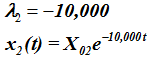System 1 is under-damped. It will display decaying oscillations in response to an initial disturbance. After 0.01 seconds, the initial disturbance is reduced by a factor = e-0.01 = 0.99 of the original value. System 1 oscillates at a frequency of 99.995 radians/sec.

In contrast, system 2 is over-damped. After 0.01 seconds, the initial disturbance is reduced by a factor = e-100 = 3.72 x 10-44 of the original value. For any initial disturbance, system response is almost instantaneously damped out. System 2 does not oscillate at all.

A system exhibiting these characteristics is called numerically stiff. One component of the solution exhibits slow decay to an initial excitation whereas another component rapidly dies almost instantaneously.

Many mechanical systems have numerically stiff properties or are characterized by differential-algebraic equations (see comment 2). These can only be solved efficiently by a stiffly-stable integrator.

2. Differential Algebraic Equations

A system of equations, which is a combination of ordinary differential equations, and algebraic constraint equations are called differential algebraic equations.

For example, in a multi-body system (MBS), Newton's second law manifests itself in the form of second order, ordinary differential equations (ODE) while constraints in the system (joints, for example) manifest themselves as algebraic equations (AE). Hence, the mathematical representation of a typical multi-body system is a system of differential algebraic equations. The mechanism shown in the figure above is usually characterized by differential algebraic equations.

Ordinary differential equations are represented as:(5)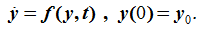y are the states to be integrated

Differential algebraic equations are represented as:

(6)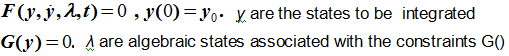3. Index of a DAE

The index of a system of DAEs is defined as the number of times you need to differentiate the constraints in the system with respect to the independent variable (usually time), to get a system of ordinary differential equations. This is illustrated in the figure below.

4. Equation Formulation
MotionSolve supports four different equation formulations for dynamic simulations:
The ODE formulation
The ODE formulation supports both stiff and non-stiff integrators. MotionSolve transforms the DAE form equations of motion into ODE form equations using coordinate partitioning and then solves the resulting equation using an ODE integrator. Both stiff and non-stiff integrators are supported. The stiff integrators supported by this formulation are (a) VSTIFF and (b) MSTIFF. The integrator ABAM is used to integrate non-stiff solutions.
The DAE Index-3 (I3) formulation
The I3 formulation provides the DAE form of the equations of motion to an integrator capable of solving this form of the equations of motion. DSTIFF is the only integrator in MotionSolve capable of solving the I3 form of the equations of motion. In the I3 formulation, the integrator does not monitor the integration local error in the velocity states. Consequently, I3 solutions typically tend to be very fast, though slightly inaccurate in velocities sometimes.
The DAE Stabilized Index-1 (SI1) formulation
The SI1 formulation provides an even more "stabilized" index-1 DAE form of the equations of motion to an integrator capable of solving this form of the equations of motion. The term "stabilized" refers to the seemingly redundant set of velocity and acceleration constraints that are added to the I3 set. These enforce consistency of velocities and accelerations with the velocity and acceleration constraints - which would be automatic if the solutions were exact. DSTIFF is the only integrator in MotionSolve capable of solving the SI1 form of the equations of motion. In the SI1 formulation, the integrator monitors the integration local error in both the displacement and velocity states. Consequently, SI1 solutions typically tend to be very accurate. The typical speed of SI1 solutions, compared to I3 solutions, is somewhat slower.
The figure below summarizes the above statements.
5. Numerical Solution
MotionSolve provides four different integration algorithms for dynamic simulations.
DSTIFF
This is the default integrator in MotionSolve. This code solves a system of differential/algebraic equations of the form G(t,y,y') = 0, using a combination of Backward Differentiation Formula (BDF) methods. This is based on the publicly available integrator DASPK. The authors of this integrator are Linda R. Petzold, Peter N. Brown, Alan C. Hindmarsh, and Clement W. Ulrich. It was developed at the Lawrence Livermore National Laboratory (LLNL) and subsequently at the University of California, Santa Barbara. This integrator is only available for the DAE forms of the equations of motion (I3 and SI1) in MotionSolve.
VSTIFF
This is a stiffly stable, fixed-leading coefficient, BDF integrator based on VODE. VODE was developed by P.N. Brown (Lawrence Livermore National Laboratories [LLNL]), G.D. Byrne (SMU), and A.C. Hindmarsh (LLNL). This is the default stiff integrator for the ODE form of the equations of motion in MotionSolve. VODE solves the initial value problem for stiff or non-stiff systems of first order ODEs of the form dy/dt = f(t,y), or, in component form, dyi/dt =fi(t,y1,y2,...,yNEQ) (i = 1,...,NEQ).
VODE
A package based on the EPISODE and EPISODEB packages, and on the ODEPACK user interface standard, with minor modifications.
MSTIFF
This is a stiffly stable, BDF integrator based on the Modified Extended Backward Differentiation Formulae (MEBDF), developed by J R Cash. This integrator is only available for the ODE form of the equations of motion in MotionSolve.
This is a non-stiff integrator, written by Laurence F. Shampine and Marilyn K. Gordon. This integrator is only available for the ODE form of the equations of motion in MotionSolve.
6. Index-3 DAE form of the Equations of Motion
Assume that a system is defined as follows:
• "N" displacement coordinates q=[q1, q2, … qN]T are used to represent the system.
• The coordinates q are not all independent.
• "M" displacement constraints Φ =[ Φ1(q,t), Φ2(q,t), … ΦM(q,t)]T exist between the "N" coordinates q.
• Let λ = [ λ1, λ2 … λM]T represent Lagrange Multipliers required to maintain the constraints Φ.
• Let F = [F1, F2 … FNa]T represent external forces acting on the system.
• Let the kinetic energy of the system be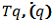and the potential energy be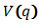.
• Then the Lagrangian function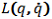may be expressed as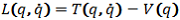.

Under these assumptions, the equations of motion for the system can be represented as: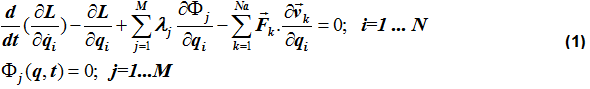The above equations are called the Euler-Lagrange representation of the equations of motion. In matrix form, equation (1) can be written as: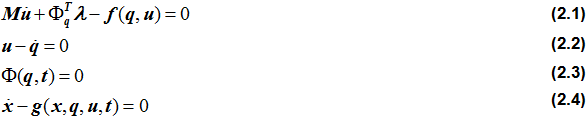Equations (2.1) represent the force balance equations. The first term represents the inertia force, the second term represents the constraint forces and the third term the generalized external forces. Equations (2.1) is thus a restatement of Newton's Second Law of Motion. Equations (2.1) are differential equations that relate the time derivative of velocity (acceleration) to the external and internal forces acting on the system.

Equations (2.2) represent the kinematic differential equations in the system. These equations represent the equations of motion in first order form. To accomplish this, a new set of variables, u, the velocities are created. Velocities are defined as first-time derivatives of displacements. Equations (2.2) are also differential equations.

Equations (2.3) represent the algebraic constraints in the system. These come into existence because the equations were not formulated in terms of an independent coordinate set. The system has p=N-M degrees of freedom. The N coordinates therefore can be related to each other through M algebraic constraint equations.

Equations (2.4) represent the user-defined differential equations, specified using Control_Diff, Control_SISO, and Control_StateEqn elements. The user-defined states are denoted by x.

Equations (2) are a set of implicit, differential-algebraic equations (DAE). Let Z = [u, q, λ, x]T. Equations (2) can be represented in compressed notation as: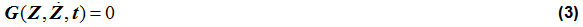By differentiating equations (2.1) once and equations (2.3) three times, you can convert equations (3) to a set of Ordinary Differential Equations (ODE) of the form: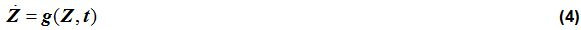The index of a DAE is defined as the number of times the constraints are to be differentiated in order to convert the DAE to a set of ODE. Since three time differentiations of the constraints (2.3) are needed to arrive at the ODE form represented by equations (4), the index of the DAE is said to be 3. Index-3 DAE are also characterized by the fact that the matrix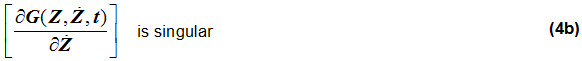A numerical solution of equations (2) or (3) requires specialized DAE integrators. These methods share the basic concept that accuracies in {u}, {q} and {λ} are controlled to different levels. One common approach (Index-3) is to ignore local integration error in {u} and {λ} and monitor local integration error in {q} only. Index-3 solutions thus tend to be less accurate, but very fast. If extremely accurate solutions are required, an alternative approach for the solution is preferred.

7. Numerical Integration of the Equations of Motion Using Stiffly-stable Integrators
The numerical integration of the equations is performed by the chosen integrator. Three stiffly-stable integrators are available today:
• VSTIFF, MSTIFF, and DSTIFF

Regardless of the integrator choice, the following three key operations are required to take a step.

a) Predict

The purpose of the predictor is to provide a good initial guess for the corrector. The predictor fits a polynomial through the previously calculated solution points. It then extrapolates the polynomial to the new value of time to "predict" the solution.

The image above shows how the predictor in DSTIFF works. Tn is the current time, and the integrator is predicting the value at tn+1. The predictor polynomial is shown in black. The extrapolation is shown in brick red. The predictor simply extrapolates past values; it does not solve the equations of motion. The order of the integrator defines the number of past values to take. In Figure 5, the order of the integrator is three, so three past values, starting from tn, are considered.

DSTIFF uses Newton Divided Differences to create an interpolating polynomial. This polynomial has the form: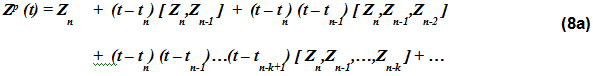The quantities [ Zn,Zn-1 ], [ Zn,Zn-1,Zn-2 ], etc. represent divided differences of the state Zn.

VSTIFF and MSTIFF use a slightly different scheme. An integrator of order K at time = tn has available to it K orders of derivatives. The integrator simply uses a Taylor's series expansion of the states Z to predict a solution at time = tn+1. This is shown in equation 8 below.

h = tn+1 - tn is the step size.

b) Correct

The purpose of the corrector is to refine the predicted so it satisfies the equations of motion:
• Equation (3) for the DAE-Index 3 formulation

Both stiff and non-stiff integrators use correctors; however, the techniques employed in the corrector differ between the two. For the Index-3 DAE formulation, DSTIFF uses a modified Newton-Raphson (N-R) method to ensure the solution satisfies the equations of motion. For more information on Newton-Raphson, please refer to the discussion in DebugOutput. To employ this method, the DAE needs to be converted to a set of nonlinear, algebraic difference equations. This transformation is explained below.

The general integration formula for stiff integrators is: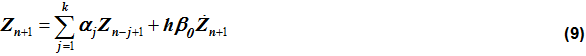In equation (9):
• h is the step size being attempted and k is the order of the integrator.
• αj, β0 are coefficients defined by the integrator. These are dependent on the method being used: VSTIFF, MSTIFF or DSTIFF.

The integration formulae converts the system of DAE's to a set of non-linear algebraic equations (NLAE) that N-R (the first phase of the corrector) can solve.

The corrector goal may be stated more precisely as:

• Given a predicted value Zn+1(p) that is determined by the predictor, find a corrected value Zn+1(c) that satisfies the equations of motion (equations 3 or 7).

Using a first order Taylor's series expansion of G() about the predicted value, you obtain,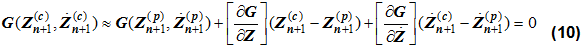Define: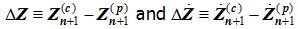Then: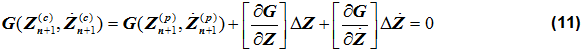From (9) we get: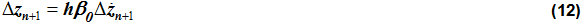Substituting (12) into (11), we get the corrector relationship: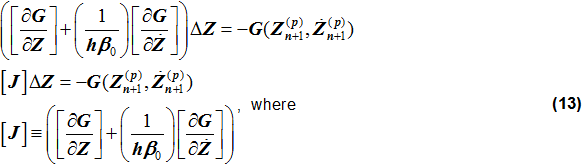The matrix [J] is called the Jacobian matrix of the equations of motion. From (13), a corrector iteration sequence is defined as shown in the figure below. At the heart of the process is the Newton-Raphson method of solving non-linear algebraic equations.

The error norm is calculated in DDWNRM(…) as shown in the figure below.

In the figure above:
• RTOL[i] (relative tolerance) and ATOL[i] (absolute tolerance) are calculated from the error tolerance, INTEGR_TOL, specified in the PARAM_TRANSIENT block of the input file and a scale factor that is dependent on the variable type: RTOL[i] = ATOL[i] = INTEGR_TOL * SCALE[i].
• SCALE[i] is calculated from the attributes VEL_TOL_FACTOR and DAE_ALG_TOL_FACTOR specified in the PARAM_TRANSIENT block of the input file and is explained further in the table below. The various states in Z are scaled as follows:
Table 1. Scale values for the solution vector.
State type Attribute Default Value for Scale
Displacement   1.0
Differential States   1.0
Velocity VEL_TOL_FACTOR 1000.0
Lagrange Multipliers DAE_ALG_TOL_FACTOR 1000.0

For displacement and user-defined differential states:

• RTOL[i] = ATOL[i] = INTEGR_TOL

For velocity states:

• RTOL[i] = ATOL[i] = VEL_TOL_FACTOR*INTEGR_TOL

For Lagrange multiplier state:

• RTOL[i] = ATOL[i] = DAE_ALG_TOL_FACTOR*INTEGR_TOL

b) Estimate local truncation error

The corrector ensures that the solution satisfies the equations of motion (EOM) (for example, the EOM are consistent), but it does not ensure accuracy. The next step in the process is calculating the integration error in the step to estimate accuracy, since it is possible that the corrected solution is not the "true" solution; rather, the prediction may have been poor, and the corrector may have found a solution that is no longer consistent with the model's initial conditions. In other words, your result may have "jumped" to a different solution in the solution manifold, albeit consistent with the EOM. The error calculation is shown in the figure below. Ck is a step-size error coefficient that is calculated by the integrator.

8. Why are stiff integrators so effective for highly-damped problems?

To understand why, consider the following test problem: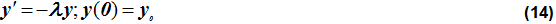The true solution for this problem is: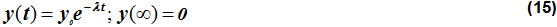The Backward Euler is a first order stiff integrator. It has the form: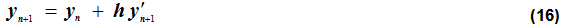Applying (16) to equation (14) yields: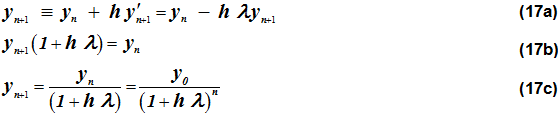As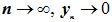for any positive value of h. Backward Euler is stable for any value of h. The larger the step-size h is, the faster yn+1 will converge to zero.

In contrast, when the same calculations are done with the Forward Euler integrator, a non-stiff integrator of order 1, the following results are seen. The Forward Euler has the form: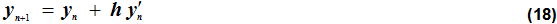Applying (18) to equation (14) yields: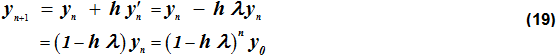Equation (19) states that for the Forward Euler:

As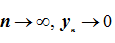only when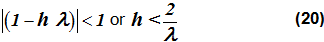Consider a highly damped problem, l=104 in equation (14).

• Equation (20) implies that the Forward Euler will yield the stable results only when the step size h < 2 x 10-4.
• Equation (17c) implies that the Backward Euler will yield stable results for any step h.

This is why stiff integrators are so effective for damped problems.

9. My model fails due to integrator failures. How can I diagnose this issue and fix it?

Multi-step integrators like DSTIFF and VSTIFF estimate the local error at each step by computing the difference between the predicted and the corrected value of the solution, and scaling it by an "error coefficient" that is calculated by the integrator. A bad prediction can therefore cause an integration error.

Predictions can be inaccurate for two reasons:
• There is a discontinuous motion input in the system.
• There is an almost discontinuous force in the system.

The effect of such modeling is shown in figures 10a and 10b below.

Discontinuities such as those shown above can be caused by function expressions or user subroutines that use IF-THEN-ELSE logic carelessly. Modeling elements that should be examined for discontinuities or sharp changes include:
• Forces
• Variables
• User Differential Equations
• General State Equations

It is a good idea to replace IF-THEN-ELSE logic with smooth STEP functions. Also, use the MotionSolve XML statement:

<DebugOutput>
SwitchOn="True"
</DebugOutput>

to enable detailed Newton-Raphson iteration output. By looking at this output, you can usually backtrack to the modeling elements that are likely causing the failure.

The normal fix for such situations is the following:

• Make sure stiffness/damping coefficients are realistic.
• Make sure masses and inertias are physically meaningful.
• Identify and eliminate discontinuity in modeling element[s].
• Avoid very steep STEP functions.
• Temporarily loosen INTEGR_TOL to understand true cause.
10. Have my results converged? That is, have they stopped changing with the error tolerance?
We recommend that you perform a convergence study to ensure that the results you get from the integrator have stopped changing (are converged). Since the integrator is approximating an exact solution, it is possible that allowing too much error in the integrator causes the integrator to provide a different result than if the error tolerance is tighter (smaller). There are a range of settings to allow you to tune the integrator as desired, but the few to start investigating first are the following:
• integr_tol
• h_max
• vel_tol_factor for DSTIFF, which requires SI1 and via the dae_index parameter)
You may get converged results in different ways, but below is one example that may work for you:
• Pick the channels of data you are interested in the model (displacements, forces, and so on).
• Pick the integrator - what does it perform error control on? (displacements, velocities, differential states).
• Reduce the error until the states that the integrator controls converge. Then, take the largest error that gives the converged result.
• If you're still interested in the states that the integrator does not control with error tolerance, reduce the integrator maximum step size (h_max) until those states converge. Then, use the largest h_max that gives the converged results.
• If the simulation fails, you can loosen (increase) error tolerance and control accuracy with the integrator maximum step size (h_max) to try to get past the problem. This will likely take more time than for a variable-step integrator, so use as a last resort.
• Many other integrator settings are also available, so consider exploring other options that may be applicable to your model.
11. Relative Comparison of the Various Formulation Approaches
The table below summarizes the relative strengths of the different DAE approaches. The following criteria are used to characterize the different approaches:
Accuracy
Measures the integration error (calculated as shown in Figure Figure 5) that is accumulated in the solution array.
Speed
Measures the relative CPU time commonly seen for the different approaches. This is highly model-dependent, and reflects our experiences to data in testing the various approaches.
Handling Discontinuities
Measures the ability of the formulation to deal with a sharp corner or a discontinuity in a system input (Motion or Force). Discontinuities and sharp corners (non-differentiability) should be avoided in models.
Consistency
Measures how well the solution satisfies the constraints Φ and its first and second time derivatives.
Tracking High Frequencies
Measures how well high frequencies in a system are tracked.
Numerical Damping
Measures the numerical damping applied because of the implementation of a certain index formulation. In general, high numerical damping leads to faster and more robust solutions, though at the expense of some accuracy.
Robustness
The default DE formulation is Index-3. We recommend that you use the Index-3 formulation first, and consider switching to other formulations only if a solution is found that lacks in the criteria shown above.
Note: The table below contains subjective information, and should therefore be regarded as a general guideline, not an absolute recommendation.
Table 2. Relative merits of the different equation formulations.
I-3 SI-1 ODE (SI-0) ABAM
Accuracy Medium High High High
Speed High Medium Low High /

Very Low*

Handling Discontinuities High Low Low High
Consistency Low High High High
Tracking High Frequencies Low High High High
Numerical Damping High Low Low Low
Robustness High Medium Medium High

(*For ABAM, Speed is high for non-stiff and very low for stiff problems)

12. It is almost always not a good idea to increase DAE_CONSTR_TOL above 0.001 mm. This tolerance governs the minimum solution accuracy for the kinematic constraints in the system specified in the length units of the model. When DAE_CONSTR_TOL is large (greater than 0.001 mm), the system constraints can be only loosely satisfied. In addition to creating inaccurate answers, errors in constraint satisfaction can also cause the numerical solution to become unstable and even cause failures.
13. The dae_jacob_eval and dae_jacob_init both control when the Jacobian is evaluated during the corrector iterations. The key difference between the two is in the way they affect the Jacobian evaluation frequency.
Let M be the iteration counter for the Newton Raphson iterations. Note that his index is implemented as a 0-index, meaning that it starts from 0. The Jacobian is re-evaluated whenever either or both of the following conditions are true:
• MOD(M, dae_jacob_eval) = 0
• M < dae_jacob_init

The following tables illustrate this better

 Corrector iteration counter (M) dae_jacob_eval = 2 dae_jacob_init = 2 New Jacobian? MOD (M, 2) = 0? M < 2? 0 Yes Yes Yes 1 No Yes Yes 2 Yes No Yes 3 No No No 4 Yes No Yes
 Corrector iteration counter (M) dae_jacob_eval = 3 dae_jacob_init = 2 New Jacobian? MOD (M, 3) = 0? M < 2? 0 Yes Yes Yes 1 No Yes Yes 2 No No No 3 Yes No Yes 4 No No No 5 No No No 6 Yes No Yes 7 No No No
14. References
VSTIFF:
• "VODE, a Variable-Coefficient ODE Solver", P. N. Brown, G. D. Byrne, and A. C. Hindmarsh. SIAM J. Sci. Stat. Comput., 10, pp1038-1051, 1989.
• "A Poly-algorithm for the Numerical Solution of Ordinary Differential Equations," G. D. Byrne and A. C. Hindmarsh, ACM Trans. Math. Software, 1 (1975), pp. 71-96.
• "EPISODE: An Effective Package for the Integration of Systems of Ordinary Differential Equations," A. C. Hindmarsh and G. D. Byrne, LLNL Report UCID-30112, Rev. 1, April 1977
• "ODEPACK, a Systematized Collection of ODE Solvers," in Scientific Computing, A. C. Hindmarsh; R. S. Stepleman et al., eds., North-Holland, Amsterdam, 1983, pp. 55-64.
DSTIFF:
• "The Numerical Solution of Initial Value Problems in Differential-Algebraic Equations", L. Petzold, K. E. Brenan and S. L. Campbell, Elsevier Science Publishing Co., (1989) , second edition, SIAM Classics Series, 1996.
• "Consistent Initial Condition Calculation for Differential-Algebraic Systems ", P. N. Brown, A. C. Hindmarsh, and L. R. Petzold, SIAM J. Sci. Comp. 19 (1998), pp. 1495-1512.
MSTIFF:
• "The Integration Of Stiff Initial Value Problems In O.D.E.S Using Modified Extended Backward Differentiation Formulae", J. Cash, Comp. and Maths. with Applics., 9:645-657, 1983.
• "The Integration Of Stiff Initial Value Problems In O.D.E.S Using Modified Extended Backward Differentiation Formulae", J.R. Cash, Comp. And Maths. With Applics., 9, 645-657, (1983).
• "Stable Recursions With Applications To The Numerical Solution Of Stiff Systems", J.R. Cash, Academic Press.(1979)
• "Ordinary Differential Equation System Solver", A.C. Hindmarsh, Gear, C.W., Ucid-30001 Rev. 3, Lawrence Livermore Laboratory, Livermore, Ca 94550, Dec. 1974.
• "An Mebdf Code For Stiff Initial Value Problems", J.R. Cash And S. Considine, ACM Transactions On Mathematical Software, 1992.

ABAM:

• "Computer Solution of Ordinary Differential Equations", Lawrence F. Shampine and Marilyn K. Gordon, W.H.Freeman & Co Ltd, June 1975.